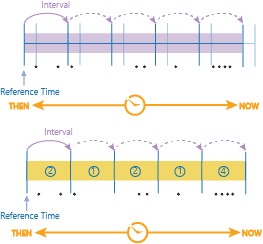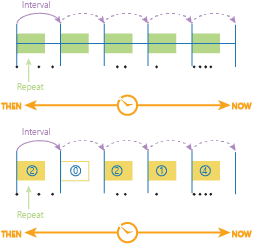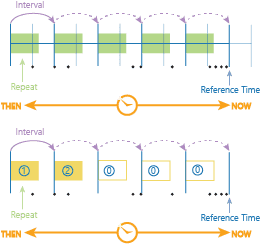# Time Stepping

GeoAnalytics Engine offers multiple tools that allow you to analyze your data using time. There are two ways to analyze using time in GeoAnalytics Engine analysis:

Time steps slice your input records into steps defined by three time step parameters (time step interval, time step repeat interval, and time step reference). When you apply time steps, analysis is completed on each time step independent of data outside the time step of interest.

The output of time steps results in a time-enabled DataFrame with fields representing the start time (`START_DATETIME`) and end time (`END_DATETIME`) of the time step interval. Using the start and end fields you can visualize your results for each time step. It is recommended to only visualize one time step at a time to avoid results drawing on top of each other.

## Time step summary

Time steps are a way of examining and analyzing your data through specified time intervals. Using three parameters, time interval, time step repeat interval, and reference time, you can specify the time steps you are interested in analyzing:

• Time step intervalThe duration of the step you are interested in analyzing.
• Time step repeat intervalHow frequently you measure the time step interval.
• Reference timeWhere in time to align the time step interval and time step repeat to, with time steps continuing both forward and backward from the reference time.

How these three parameters work together is explained below using examples.

## Examples

### Time step interval only

The most basic time step is only specified by a time step interval (for example, 1 hour). In this example, these steps would result in four time steps. The slicing is automatically aligned at January 1, 1970, and steps are calculated from that point. Time steps that are empty do not return any results.

### Time step interval and reference time

If you want to specify a time for the time steps to begin (instead of the default January 1, 1970), you can specify a reference time. A reference time can be a date (January 1, 2016) or a time and date (January 1, 2016, at 9:30 a.m.). You cannot only apply a time (9:30 a.m.). When you apply a reference time, the time steps shift from the previous example and align with the reference time (the new steps are in dark blue, and the previous are in light blue).

This example has different results due to the reference time being used.Time stepping that provides a time step interval aligned to a given reference time. The light blue indicates the time steps from the time step interval only example.

### Time step interval and time step repeat interval

When using a time step interval without a repeat, the duration of your time step will always be equal to the time step. You can set a different time step repeat interval and time step interval so that analysis is completed with breaks between the time steps. For example, if you did a time step interval of 1 day, and a time step repeat interval of 1 hour, you would get the first hour of the day (that starts at the default reference time of January 1, 1970).

In this example, there are four time steps (yellow time steps with blue numbers).Time stepping using a step interval and time step repeat. Here the repeat is shorter than the time step interval, and no reference time is specified. An example of this could be time step interval = 1 month, time step repeat = 1 week. This would be the first week of the month.

### Time step interval, time step repeat, and reference time

Using all three parameters gives you the most control of your time steps. You can specify how often to analyze on time, how long, and what your steps are aligned to.Time stepping that applies a time step interval, a time step repeat, and a reference time.Above is the old time alignment (light blue without arrows) that was determined by the default time start, and the new, user-defined alignment, determined by the reference time. The time steps and intervals have again shifted, and the results are different from previous examples. For another example of what you can do, if you wanted to look at trends for data points that occurred every Monday, you would set the following parameters:Data points from every Monday.
• Time step interval: 1 week
• Time step repeat interval: 1 day
• Reference time: Monday, January 1, 2016.

## Time step and interval inputs

Time steps and intervals are input as string. The recognized time types are as follows:

• Milliseconds
• Seconds
• Minutes
• Hours
• Days
• Weeks
• Months
• Years

## Time stepping results

When you analyze your data and apply time stepping, your output will be in the same geometry type (point, polyline, polygon) as it would if time wasn't enabled. Time stepping always results in a time interval, which is represented by two fields in your output DataFrame, start_time and end_time. You can view your complete dataset without accounting for time, but you may have overlapping geometries.